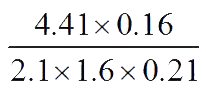# Sample Aptitude Questions of JP-Morgan

1. A number is such that when it is multiplied by ‘8’, it gives another number which is as much more than 153 as the original number itself is less than 153. What is 25% of the original number?
1. 8
2. 7.5
3. 10
4. 8.5
5. 6.5

Let the no. be x
Given 8x-153=153-x
Hence x=34
25% of x=8.5
2. A and B can complete a piece of work in 80 days and 120 days respectively. They started working together but A left after 20 days. After another 12 days C joined B and they completed the work in 28 more days. In how many days can C alone complete the work?
1. 110 days
2. 112 days
3. 114 days
4. 120 days
5. None of theseSo efficiency of A and B are 3 units and 2 units respectively.
As they worked for 20 days together after that A left so total unit contribution in 20 days= (3+2) units * 20 days = 100 units
Remaining units = 240 – 100 = 140 units
After another 12 days C joined B and they completed the work in 28
more days, so total units contribution of B in 40 days = 2*40 = 80 units
Remaining units i:e = 140-80 = 60 units
Now 60 units is done by C in 28 days
So to do 240 units C require = 28/60 *240 = 112 days
1. The HCF and LCM of two numbers are 12 and 924 respectively. Then the number of such pairs is
1. 0
2. 1
3. 2
4. 3
5. 4

Let the numbers be 12x and 12y where x and y are prime to each other.
∴ LCM = 12xy
∴ 12xy = 924
=> xy = 77
∴ Possible pairs = (1,77) and (7,11)
2. What is the least number which, when divided by 5, 6, 7, 8 gives the remainder 3 but is divisible by 9?
1. 1463
2. 1573
3. 1683
4. 1793
5. None of these

LCM of 5, 6, 7, 8 = 35 × 24 = 840
∴ Required number = 840 k + 3 which is exactly divisible by 9.
For k = 2, it is divisible by 9.
∴ Required number = 840k + 3
= 840 × 2 + 3 = 1683
3. By walking at of his usual speed, a man reaches his office 20 minutes later than his usual time. The usual time taken by him to reach his office is:
1. 75 minutes
2. 60 minutes
3. 40 minutes
4. 30 minutes
5. 20 minutes

4/3 of usual time = Usual time + 20 minutes
1/3 of usual time
= 20 minutes
Usual time = 20 × 3 = 60 minutes
4.is simplified to:
1. 1
2. 0.1
3. 0.01
4. 10
5. 0.001

4.41 x 0.16 / 2.1 x 1.6 x 0.21 =441 x 16 / 21 x 16 x 21 =1
5. By selling a bicycle for Rs. 2,850, a shopkeeper gains 14%. If the profit is reduced to 8%, then the selling price will be:
1. Rs. 2,600
2. Rs. 2,700
3. Rs. 2,800
4. Rs. 3,000
5. Rs.3,500

CP of bicycle = 100/114 x 2850 =Rs 2500
SP for a profit of 8% = 108/100 x 2500 = 2700
6. By selling an article, a man makes a profit of 25% of its selling price. His profit per cent is:
1. 20
2. 25
3. 16
4. 15
5. 33 1/3

If the SP of article be Rs. x then its CP is x - x/4 =Rs. 3x/4
∴ Gain % = (x/4) / (3x/4) x 100 = 100/3 = 33 1/3 %
7. Monthly incomes of A and B are in the ratio of 4 : 3 and their expenses bear the ratio 3:2. Each of them saves Rs. 6,000 at the end of the month, then the monthly income of A is:
1. Rs. 12,000
2. Rs. 24,000
3. Rs. 30,000
4. Rs. 60.000
5. Rs. 35,000

Let the monthly incomes of A and B be Rs. 4x and Rs. 3x respectively and their expenditures be Rs. 3y and Rs. 2y respectively.
4x – 3y = 6000 and 3x – 2y = 6000
⇒ 4x – 3y = 3x – 2y ⇒ x = y = 4x – 3y = 6000
⇒ x = 6000
⇒ A’s monthly income = 4x = Rs. 24000
8. The average of three consecutive odd numbers is 12 more than one third of the first of these numbers. What is the last of the three numbers?
1. 15
2. 17
3. 19
4. 21